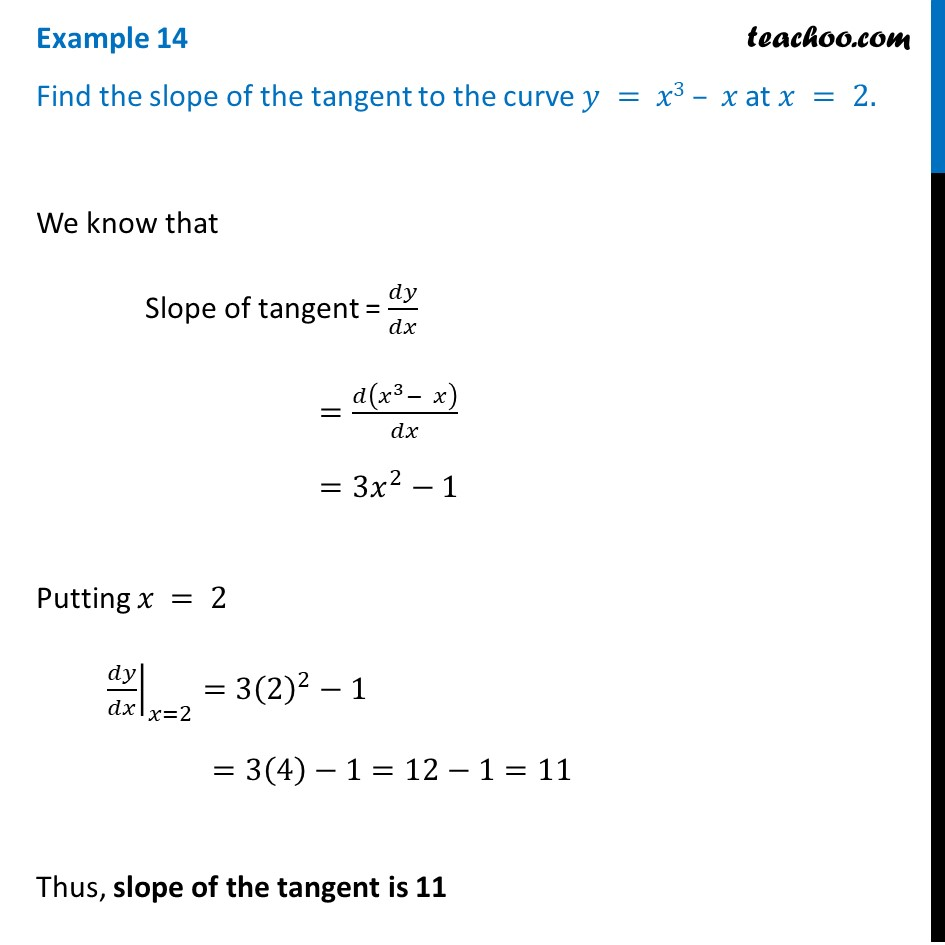Finding slope of tangent/normal

Chapter 6 Class 12 Application of Derivatives (Term 1)
Concept wise### Transcript

Example 14 Find the slope of the tangent to the curve 𝑦 = 𝑥3 – 𝑥 at 𝑥 = 2.We know that Slope of tangent = 𝑑𝑦/𝑑𝑥 =𝑑(𝑥^3− 𝑥)/𝑑𝑥 =3𝑥^2−1 Putting 𝑥 = 2 ├ 𝑑𝑦/𝑑𝑥┤|_(𝑥=2)=3(2)^2−1" " =3(4)−1=12−1=11 Thus, slope of the tangent is 11